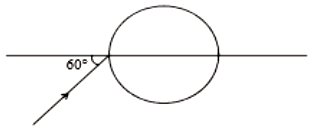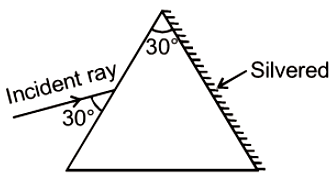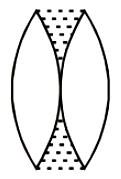A ray of light falls on a transparent sphere as shown in the figure. If the final ray emerges from the sphere parallel to the horizontal diameter, then calculate the refractive index of the sphere. Consider that the sphere is kept in the air.1.

2.

3.

4.  $\sqrt{4/3}$

Concept Questions :-

Refraction at curved surface
High Yielding Test Series + Question Bank - NEET 2020

Difficulty Level:

A small telescope has an objective lens of focal length 144 cm and an eye-piece of focal length 6.0 cm. The magnifying power of the telescope is ( when the final image is at infinity)

1.   24

2.  25

3.  30

4.  150

Concept Questions :-

Telescope
High Yielding Test Series + Question Bank - NEET 2020

Difficulty Level:

The focal length of the plano-convex lens is 20 cm. If its plane surface is silvered, then

1.  it behaves as a concave mirror of focal length 10 cm

2.  it behaves as a convex mirror of  focal length of 10 cm

3.  it behaves as a concave mirror of focal length 20 cm

4.  none of these

Concept Questions :-

Lenses
High Yielding Test Series + Question Bank - NEET 2020

Difficulty Level:

The critical angle for prism is 36$°$. The maximum angle of prism for which the emergent ray is possible is:

1.

2.

3.

4.  $144°$

Concept Questions :-

Total internal reflection
High Yielding Test Series + Question Bank - NEET 2020

Difficulty Level:

When a ray is refracted from one medium to another, the wavelength changes from 6000$\stackrel{0}{\mathrm{A}}$ to 4000$\stackrel{0}{\mathrm{A}}$. The critical  angle for the interface will be:

1.

2.

3.

4.  ${\mathrm{cos}}^{-1}\left(\frac{2}{\sqrt{3}}\right)$

Concept Questions :-

Refraction at plane surface
High Yielding Test Series + Question Bank - NEET 2020

Difficulty Level:

The sun (diameter D) subtends an angle $\mathrm{\theta }$ radian at the pole of a concave mirror of focal length f. The diameter of the image of the sun formed by the mirror is:

1.

2.

3.

4.  $\mathrm{D\theta }$

Concept Questions :-

Reflection at spherical surface
High Yielding Test Series + Question Bank - NEET 2020

Difficulty Level:

In the situation shown, the incident monochromatic ray retraces its path after its incidence on the silvered surface. The speed of light inside the prism will be1.

2.

3.

4.

Concept Questions :-

Prisms
High Yielding Test Series + Question Bank - NEET 2020

Difficulty Level:

If the wavelength of light used is halved and the numerical aperture of the compound microscope is doubled, then its resolving power will

1. Remain unchanged

2. Doubled

3. Halved

Concept Questions :-

Resolution of Optical devices
High Yielding Test Series + Question Bank - NEET 2020

Difficulty Level:

The length of an astronomical telescope adjusted for parallel light is 90 cm. If the magnifying power of the telescope is 17, then the focal length of eyepiece and objective are respectively

1.  10 cm and 80 cm

2.  85 cm and 5 cm

3.  70 cm and 20 cm

4.  5 cm and 85 cm

Concept Questions :-

Telescope
High Yielding Test Series + Question Bank - NEET 2020

Difficulty Level:

Two identical equiconvex thin lenses each of focal lengths 20 cm, made of material of refractive index 1.5 are placed coaxially in contact as shown. Now, the space between them is filled with a liquid of refractive index 1.5. The equivalent power of this arrangement will be:1.  + 5D

2.  Zero

3.  + 2.5D

4.  + 0.5D

Concept Questions :-

Lensmakers' formula